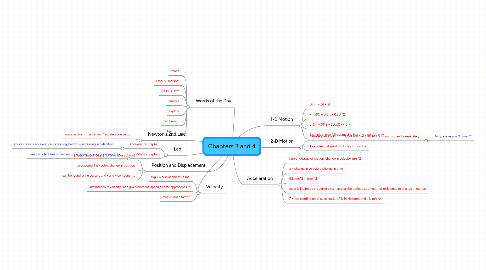# Chapters 3 and 4

Get Started. It's FreeChapters 3 and 4## 5. Lab

### 5.1. Acceleration graphs

5.1.1. above x-axis is acceleration increasing, below is decreasing acceleration

### 5.2. Velocity graphs

5.2.1. parabolic identifies movement; open down is -a and open up is +a

## 8. 2-D Motion

### 8.1. same equations as for 1-D, but with velocity and position for x and y separately

8.1.1. for y, a always is 9.8 m/s^2